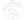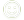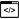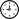Courses Details
Analyzing Big Data with Microsoft RCourse Code 20773Duration 3 Days
Request Group Training
Course Overview
The main purpose of the course is to give students the ability to use Microsoft R Server to create and run an analysis on a large dataset, and show how to utilize it in Big Data environments, such as a Hadoop or Spark cluster, or a SQL Server database.
Course Schedule
Target Audience
The primary audience for this course is people who wish to analyze large datasets within a big data environment.
The secondary audience are developers who need to integrate R analyses into their solutions.
Course Prerequisites

In addition to their professional experience, students who attend this course should have:

• Programming experience using R, and familiarity with common R packages
• Knowledge of common statistical methods and data analysis best practices.
• Basic knowledge of the Microsoft Windows operating system and its core functionality.

Working knowledge of relational databases.

Expected Accomplishments

After completing this course, students will be able to:

• Explain how Microsoft R Server and Microsoft R Client work
• Use R Client with R Server to explore big data held in different data stores
• Visualize data by using graphs and plots
• Transform and clean big data sets
• Implement options for splitting analysis jobs into parallel tasks
• Build and evaluate regression models generated from big data
• Create, score, and deploy partitioning models generated from big data
• Use R in the SQL Server and Hadoop environments
Course Outline
Module 1: Microsoft R Server and R ClientExplain how Microsoft R Server and Microsoft R Client work.Lessons
• What is Microsoft R server
• Using Microsoft R client
• The ScaleR functions
Lab : Exploring Microsoft R Server and Microsoft R Client
• Using R client in VSTR and RStudio
• Exploring ScaleR functions
• Connecting to a remote server

After completing this module, students will be able to:

• Explain the purpose of R server.
• Connect to R server from R client
• Explain the purpose of the ScaleR functions.
Module 2: Exploring Big DataAt the end of this module the student will be able to use R Client with R Server to explore big data held in different data stores.Lessons
• Understanding ScaleR data sources
• Reading data into an XDF object
• Summarizing data in an XDF object
Lab : Exploring Big Data
• Reading a local CSV file into an XDF file
• Transforming data on input
• Reading data from SQL Server into an XDF file
• Generating summaries over the XDF data

After completing this module, students will be able to:

• Explain ScaleR data sources
• Describe how to import XDF data
• Describe how to summarize data held in XCF format
Module 3: Visualizing Big DataExplain how to visualize data by using graphs and plots.Lessons
• Visualizing In-memory data
• Visualizing big data
Lab : Visualizing data
• Using ggplot to create a faceted plot with overlays
• Using rxlinePlot and rxHistogram

After completing this module, students will be able to:

• Use ggplot2 to visualize in-memory data
• Use rxLinePlot and rxHistogram to visualize big data
Module 4: Processing Big DataExplain how to transform and clean big data sets.Lessons
• Transforming Big Data
• Managing datasets
Lab : Processing big data
• Transforming big data
• Sorting and merging big data
• Connecting to a remote server

After completing this module, students will be able to:

• Transform big data using rxDataStep
• Perform sort and merge operations over big data sets
Module 5: Parallelizing Analysis OperationsExplain how to implement options for splitting analysis jobs into parallel tasks.Lessons

• Using the RxLocalParallel compute context with rxExec
• Using the revoPemaR package
Lab : Using rxExec and RevoPemaR to parallelize operations
• Using rxExec to maximize resource use
• Creating and using a PEMA class

After completing this module, students will be able to:

• Use the rxLocalParallel compute context with rxExec
• Use the RevoPemaR package to write customized scalable and distributable analytics.
Module 6: Creating and Evaluating Regression ModelsExplain how to build and evaluate regression models generated from big dataLessons
• Clustering Big Data
• Generating regression models and making predictions
Lab : Creating a linear regression model
• Creating a cluster
• Creating a regression model
• Generate data for making predictions
• Use the models to make predictions and compare the results

After completing this module, students will be able to:

• Cluster big data to reduce the size of a dataset.
• Create linear and logit regression models and use them to make predictions.
Module 7: Creating and Evaluating Partitioning ModelsExplain how to create and score partitioning models generated from big data.Lessons
• Creating partitioning models based on decision trees.
• Test partitioning models by making and comparing predictions
Lab : Creating and evaluating partitioning models
• Splitting the dataset
• Building models
• Running predictions and testing the results
• Comparing results

After completing this module, students will be able to:

• Create partitioning models using the rxDTree, rxDForest, and rxBTree algorithms.
• Test partitioning models by making and comparing predictions.
Module 8: Processing Big Data in SQL Server and HadoopExplain how to transform and clean big data sets.Lessons
• Using R in SQL Server